CLAT  >  Power and Index

# Power and Index - Notes | Study Quantitative Techniques for CLAT - CLAT

 1 Crore+ students have signed up on EduRev. Have you?

Chapter 2 Power and Index

In pn, p is called the base and n is called the power or index
In (-5)7, -5 is te base and 7 is the power
In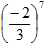,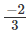is the base and 7 is the power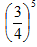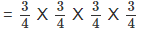And=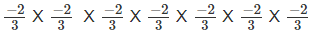Remarks: In the exponential notation, the base can be any rational number and the power can be any integer.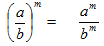Note: If the power of a rational number is 1 its value will be the rational number itself
i.e. (-10)1 = -10,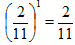and in general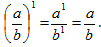.

Example: Find the value of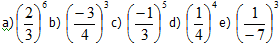Solution: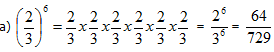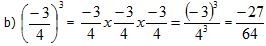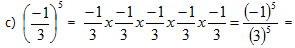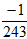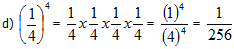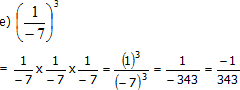Product law for exponents: If p is a non-zero rational number and m and n are two positive integers then pm x pn = p m+n
also pm x pn x pr x ps = pm+n+r+s
also (pm)n = pmn

Quotient law for exponents: It p is a non- zero rational number and m and n are two positive integers
then pm ÷   pn = pm-n   for m > n
and pm ÷ pn =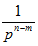for m < n

If power is zero (o): If p is a non-zero rational number then po = 1
If power is (-1): If p is a non-zero rational number then p-1 denotes the reciprocal of p and (p)-1 =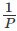A negative integer as power
p-m =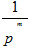other laws of exponents
pm x qm = (p x q)m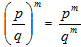Few examples showing the application of laws of exponents.
Example1: Simplify (a)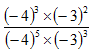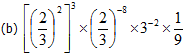Solution: a)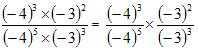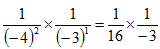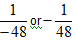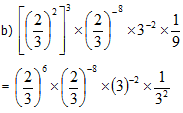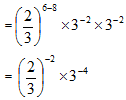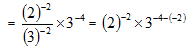= 2-2 x 3-4+2 = 2-2 x 3-2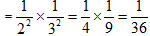Example2: Find m if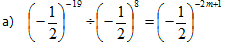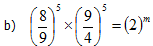Solution
(a) LHS=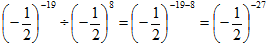RHS =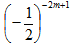Equating LHS and RHS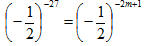Because base is same, powers must be equal
So -2m + 1 = -27
or -2m  = -27 – 1
= -28
or  m  = 14
(b) LHS =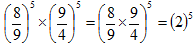RHS = 2m
So 2m = 25
Or m = 5.

Example: Solve for x
a) 3x = 81              b) (72x)-2 = (2401)
-1

Solution a) RHS = 81 = 34
So 3x = 34 or x = 4
Solution b) RHS= (2401)-1 = (74)-1 = 7-4
LHS = (72x)-2 = 7-4x
Equating LHS and RHS
7-4X = 7-4
Base in same, powers must be equal
-4x = -4
Or  x = 1

What is the difference between exponents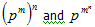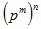= pm x pm x pm x pm --------- n times
=pm+m+m+m ------ n times
=pm n
Where as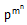=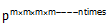Let us simplify it with the help of an example
Find the difference between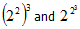(22)3 = 22 x 22 x 22 = 26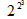= 22x2x2 = 28
So the diff. is very clear.

The document Power and Index - Notes | Study Quantitative Techniques for CLAT - CLAT is a part of the CLAT Course Quantitative Techniques for CLAT.
All you need of CLAT at this link: CLAT

## Quantitative Techniques for CLAT

56 videos|35 docs|91 tests
 Use Code STAYHOME200 and get INR 200 additional OFF

## Quantitative Techniques for CLAT

56 videos|35 docs|91 tests

Track your progress, build streaks, highlight & save important lessons and more!

,

,

,

,

,

,

,

,

,

,

,

,

,

,

,

,

,

,

,

,

,

;